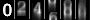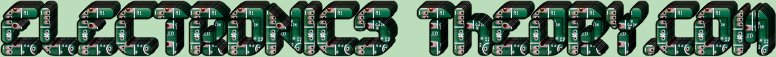# Characteristics of a Sine Wave

As stated before, by graphing the current vs. time, we end up with a pattern known as a SINUSOIDAL WAVE, or SINE WAVE for short. We say that the sine wave has positive and negative peaks at 90o and 270o respectively.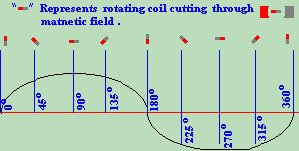A sine wave has several important characteristics.
1. One complete revolution of a generator, from 0o to 360o, is known as a CYCLE.
2. The DISTANCE between the beginning of one cycle, and the beginning of the next cycle is called one WAVELENGTH. The symbol for WAVELENGTH in electronics is the Greek letter lambda (l).
3. The TIME in which it takes to complete one cycle is known as a PERIOD.
4. The number of complete sine wave cycles generated in one second, is called FREQUENCY, and is measured in CYCLES PER SECOND (cps), PERIODS PER SECOND (pps) or more often HERTZ(Hz).
5.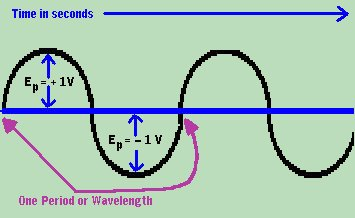6. The height of the sine wave is called the AMPLITUDE, and is measured in Voltage. The highest point of any wave is called the PEAK AMPLITUDE or PEAK VOLTAGE.
7. The difference in amplitude between the highest positive voltage, and the highest negative voltage is called the PEAK TO PEAK VOLTAGE, which is equal to twice the peak Voltage.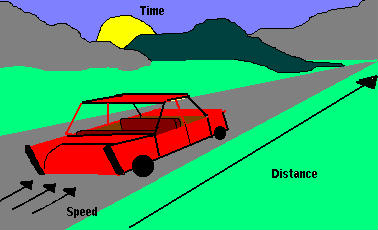Now without going into a whole bunch of math and physics...(yea, RIGHT!)... you learned sometime in your life that there is a relationship between distance, time, and speed. If you are driving down a road at 50 miles per hour, for exactly one hour, you know from basic math that you will have traveled exactly 50 miles. Why?

Distance = Speed x Time

This is a basic law of physics. It also applies to electricity and waves.

If a Wavelength is a length (read: Distance) then we can use time to compare the speed at which it travels through space. Now electricity travels at the speed of light, but what exactly is that? Well, it differs slightly from one medium to another, but for our purposes, we will assume the speed of light in a vacuum.

Wavelength = Speed x Time
We know that
1. Speed of light = 299,792,458 meters/second (In a perfect vacuum)

2. Time = (299,792,458) Speed / Distance (l )
If we assume a signal with a 2 meter long wavelength, we can use the following formula to find the time that it takes to complete one cycle:Using this formula we can plug in the numbers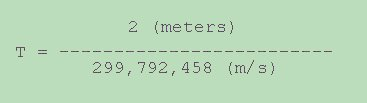Now by simple division, we get the following answer:Now if a 2 meter long signal is equal to 6.67 nanoseconds, we can find the frequency of the signal as follows: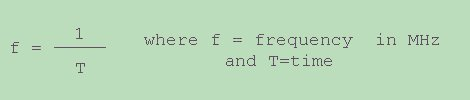Let's plug in the numbers...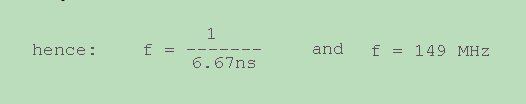Since we know that 149MHz = 2 meters in length, we can deduce that frequency and wavelength have a relationship such that:

Wavelength (in meters) = 298 / Frequency (in MHZ)

or if you prefer: Wavelength (in feet) = 936 / Frequency (in MHZ)

Let us test this theory. The 11 meter band, otherwise known as the CB Radio, or Citizen's Band, resides at (approx.) 27 MHZ. Does the math work out?The formulas you can look up in the future, should you need them. The basic knowledge we have tried to stress here is, that WAVELENGTH is a DISTANCE, a PERIOD is an amount of TIME, and that FREQUENCY is the number of wavelengths in a second. Furthermore, we stressed that all of these terms are related, but not quite interchangeable.
 (On The Following Indicator... PURPLE will indicate your current location) 1 2 3 4 5 6 7 8 9 10 11 12 13 14 15 16 17 18 19 20 21 22 23 24 25 26 27 28 29 30 31 32 33 34 35 36 37 38 39 40 41 42 43 44 45 46 47 48 49 50 51 52 53 54 55 56 57 58 59 60 61 62 63 64 65 66 67 68 69 70 71 72 73 74 75

[COURSE INDEX] [ELECTRONICS GLOSSARY] [HOME]Otherwise - please click to visit an advertiser so they know you saw their ad!

VISITORS: